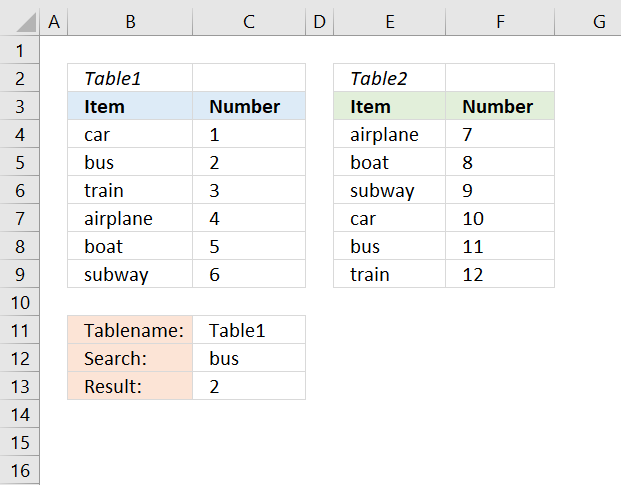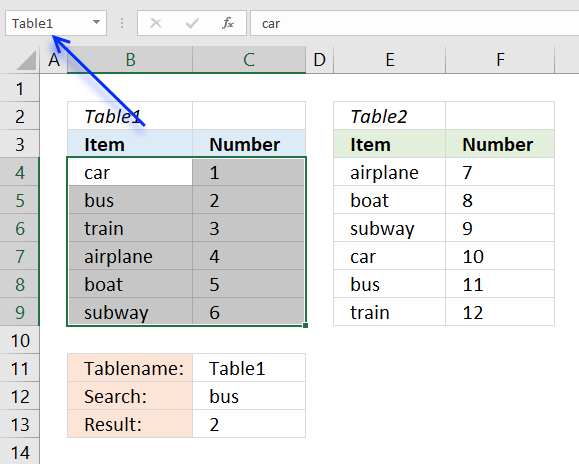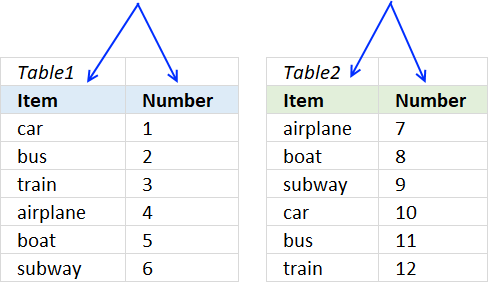Author: Oscar Cronquist Article last updated on August 10, 2018Question: How do I search a specific data set, I have two tables to choose from?

Formula in cell C13:

=VLOOKUP(C12,INDIRECT(C11),2,0)

### Define named rangesYou also need to define the data sets as named ranges.

1. Select cell range B4:C9
2. Type in the name box the name you want to use. I wrote Table1.

Repeat above steps with the second data set, I named it Table2. You may define more named ranges if you like.

### Dataset layout

Make sure that the tables have data arranged the same way, the VLOOKUP function gets the value from column 2 in the example above.If you don't have the columns arranged in the same way in both tables you will get the wrong value.

### Explaining formula

#### Step 1 - Convert named range to a cell reference

The VLOOKUP function can't evaluate a text string in the formula, however, the INDIRECT function lets you do that.

INDIRECT(C11)

becomes

INDIRECT("Table1")

and returns B4:C9.

Keep in mind that the INDIRECT fiunction is volatile and may slow down your workbook considerably if used extensively.

#### Step 2 - Get the correct value from a given column number based on a lookup value

VLOOKUP(C12,INDIRECT(C11),2,0)

becomes

VLOOKUP(C12,B4:C9,2,0)

becomes

VLOOKUP("bus",B4:C9,2,0)

and returns 2 in cell C13.

### Get Excel *.xlsx file

Choose between two data sets to VLOOKUP.xlsx